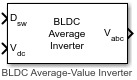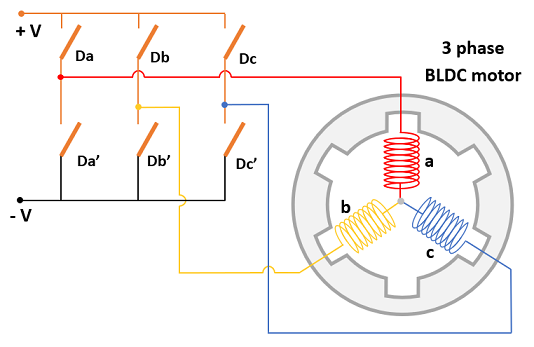# BLDC Average-Value Inverter

Compute three-phase trapezoidal AC voltage from inverter DC voltage

Since R2023a

•Libraries:
Motor Control Blockset / Electrical Systems / Inverters

## Description

The BLDC Average-Value Inverter block models an average-value and full-wave inverter. It computes the three-phase trapezoidal voltage output from the inverter DC voltage by using the six duty cycles corresponding to six inverter switches.### Equations

These equations describe how the block computes the three-phase AC voltage.

`${V}_{a}={V}_{dc}×\left({D}_{a}-{D}_{a\text{'}}\right)$`
`${V}_{b}={V}_{dc}×\left({D}_{b}-{D}_{b\text{'}}\right)$`
`${V}_{c}={V}_{dc}×\left({D}_{c}-{D}_{c\text{'}}\right)$`

where:

• Da, Db, Dc, Da', Db', and Dc' are the duty cycles (ranging between 0 and 1) corresponding to the six switches of the inverter (shown in the figure).

• ${V}_{a}$, ${V}_{b}$, and ${V}_{c}$ are the output three-phase trapezoidal voltages (volts).

• Vdc is the DC bus voltage of the inverter (volts).

## Ports

### Input

expand all

Six duty cycles in the range [0, 1] for generating the three-phase voltages that run the motor.

Data Types: `single` | `double` | `fixed point`

DC bus voltage input to the inverter.

Data Types: `single` | `double` | `fixed point` | `uint8` | `uint16` | `uint32`

### Output

expand all

Three-phase trapezoidal voltage (Volts) corresponding to the input duty cycles that runs the motor.

Data Types: `single` | `double` | `fixed point`

## Version History

Introduced in R2023a